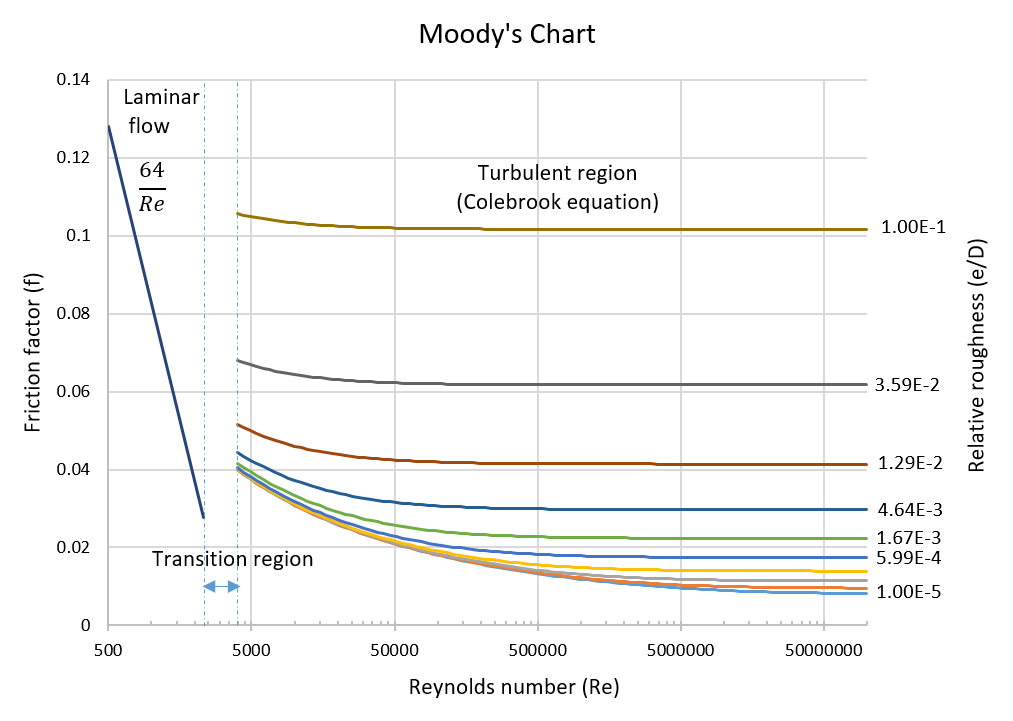# Darcy friction factor = f

@wikipedia

Quantitative dimensionless measure $//$ of the friction forces between fluid flow and inner pipe walls based on Darcy–Weisbach equation.

It depends on Reynolds number $//$ and inner pipe walls roughness $//$  (see Fig. 1): $//$.Fig. 1. Schematic chart showing how Darcy friction factor depends on Reynolds number $//$ and roughness $//$ (following )

In engineering practice one can use either Moody Chart or Darcy friction factor @model to estimate the actual value of Darcy friction factor.

Darcy friction factor $//$  takes only positive values $// 0\end{array} //]]>$ but has singularity at zero flow velocity: $//$ which may cause computational challenges.

Using Reduced Friction Factor $//$  instead can help in computations as it stays finite for all finite values of Reynolds number $//$